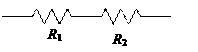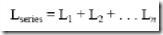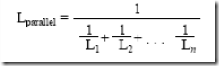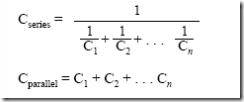### Electric Device and Electron Device–Circuit Analysis Techniques (Unit 1) Lectures

Anna University

Electric Circuit and Electron Device

UNIT-I

CIRCUIT ANALYSIS TECHNIQUES

Ohm’s Law

Temperature remaining constant, the potential difference (E) across the ends of a conductor is proportional to the current (I) flowing through it.

Mathematically, V=IR

Kirchhoff's Current Law (KCL)

"The algebraic sum of all currents entering and exiting a node must equal zero"

SIin = SIout

Similarly, at any instant the algebraic sum of all the currents at any circuit node is zero.
SI = 0

Kirchhoff's Voltage Law (KVL)

"The algebraic sum of all voltages in a loop must equal zero"

SE = SIZ

Similarly, t any instant the algebraic sum of all the voltages around any closed circuit is zero:
SE - SIZ = 0

Series and Parallel Resistor Combinations

There are two basic ways in which to connect more than two circuit components:

Series and Parallel.

• For analysis, series resistors/impedances can be replaced by an equivalent resistor/ impedance.

• Parallel resistors/impedances can be replaced by an equivalent resistor/ impedance.

Series Resistance

Two elements are in series if the current that flows through one must also flow through the other.

 SERIES Req = R1 + R2 + R3Req is equivalent to the resistor network on the left in the sense that they have the same i-v characteristics.

Parallel Resistance

Two elements are in parallel if they are connected between (share) the same two (distinct) end nodes.

 PARALLELInductors

Series and parallel inductancesWhere, L = Inductance in henrys

Capacitors

Series and Parallel CapacitancesWhere, C = Capacitance in farads

Mesh current method

The Mesh Current Method uses simultaneous equations, Kirchhoff's Voltage Law, and Ohm's Law to determine unknown currents in a network. It differs from the Branch Current method in that it does not use Kirchhoff's Current Law, and it is usually able to solve a circuit with less unknown variables and less simultaneous equations.

Steps to follow for the .Mesh Current method of analysis:

1. Draw mesh currents in loops of circuit, enough to account for all components.
2. Label resistor voltage drop polarities based on assumed directions of mesh currents.
3. Write KVL equations for each loop of the circuit, substituting the product IR for E in each resistor term of the equation. Where two mesh currents intersect through a component, express the current as the algebraic sum of those two mesh currents.
4. Solve for unknown mesh currents (simultaneous equations).
5. If any solution is negative, then the assumed current direction is wrong!
6. Algebraically add mesh currents to find current in components sharing multiple mesh currents.
7. Solve for voltage drops across all resistors (E=IR).

Node voltage method

The node voltage method of analysis solves for unknown voltages at circuit nodes in terms of a system of KCL equations. This analysis looks strange because it involves replacing voltage sources with equivalent current sources.

Node voltage rules:

1. Convert voltage sources in series with a resistor to an equivalent current source with the resistor in parallel.
2. Change resistor values to conductance.
3. Select a reference node(E0)
4. Assign unknown voltages (E1)(E2) ... (EN)to remaining nodes.
5. Write a KCL equation for each node 1,2, ... N. The positive coefficient of the first voltage in the first equation is the sum of conductances connected to the node. Repeat for coefficient of second voltage, second equation, and other equations. These coefficients fall on a diagonal.
6. All other coefficients for all equations are negative, representing conductances between nodes. The first equation, second coefficient is the conductance from node 1 to node 2, the third coefficient is the conductance from node 1 to node 3. Fill in negative coefficients for other equations.
7. The right hand side of the equations is the current source connected to the respective nodes.
8. Solve system of equations for unknown node voltages.

NETWORK THEOREMS:

Thevenin's Theorem:

• Any circuit with sources (dependent and/or independent) and resistors can be replaced by an equivalent circuit containing a single voltage source and a single resistor.

• Thevenin’s theorem implies that we can replace arbitrarily complicated networks with simple networks for purposes of analysis.

Norton's Theorem:

Any circuit with voltage sources, resistances (impedances) and open output terminals can be replaced by a single current source in parallel with single resistance (impedance), where the value of current source is equal to the current passing through the short circuit output terminals and the value of the resistance (impedance) is equal to the resistance seen into the output terminals.

Super Position Theorem:

In a linear, lumped element, bilateral electric circuit that is energized by two or more sources the current in any resistor is equal to the algebraic sum of the separate currents in the resistor when each sources acts separately. While one source is applied, the other sources are replaced by their respective internal resistances.

Super Position Theorem is not valid for power responses. It is applicable only for computing voltage and Current responses.

Maximum Power transfer Theorem:

The maximum Power transferred to a load resistor occurs when it has a value equal to the resistance of the network looking back at it from the load terminals (all sources being replaced by their respective internal resistances).

Duality:

Two electrical networks which are governed by the same type of equations are called duality.

For the networks to be duals it is necessary that the variables & elements of one network should also be the duals of variables & elements of other networks.

Method of drawing duality (or) dual network:

a) Place a dot in each independent loop of the given network. These dots placed inside the loops correspond to the independent node in the dual network.

b) A dot is placed outside the given network. This corresponds to the reference node of the dual network.

c) All the dots are connected by dotted lines crossing all the branches. The dotted lines should cross only one branch at a time.

d) The dual elements will form the branches connecting the corresponding nodes in the dual network.

Star-Delta Transformation:

The Star-Delta transformation techniques are useful in solving complex network.

A star network of three resistances RA, RB and RC connected together at common node N can be transformed into a delta network of three resistances RAB, RBC and RCA by the above equations:

In general terms:
Rdelta = (sum of Rstar pair products) / (opposite Rstar)

Delta-Star Transformation

A delta network of three impedances RAB, RBC and RCA can be transformed into a star network of three impedances RA, RB and RC connected together at common node N by the following equations:

In general terms:
Rstar = (adjacent Rdelta pair product) / (sum of Rdelta)